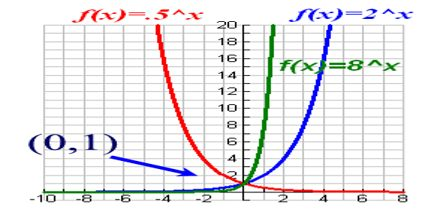Mathematic

# Exponential FunctionsExponential Functions are functions which can be represented by graphs similar to the graph on the right. This lecture briefly describe on it with examples and graph. Exponential Function is a function whose value is a constant raised to the power of the argument, especially the function where the constant is e. All base exponential functions are similar because they all go through the point (0,1), regardless of the size of their base number.